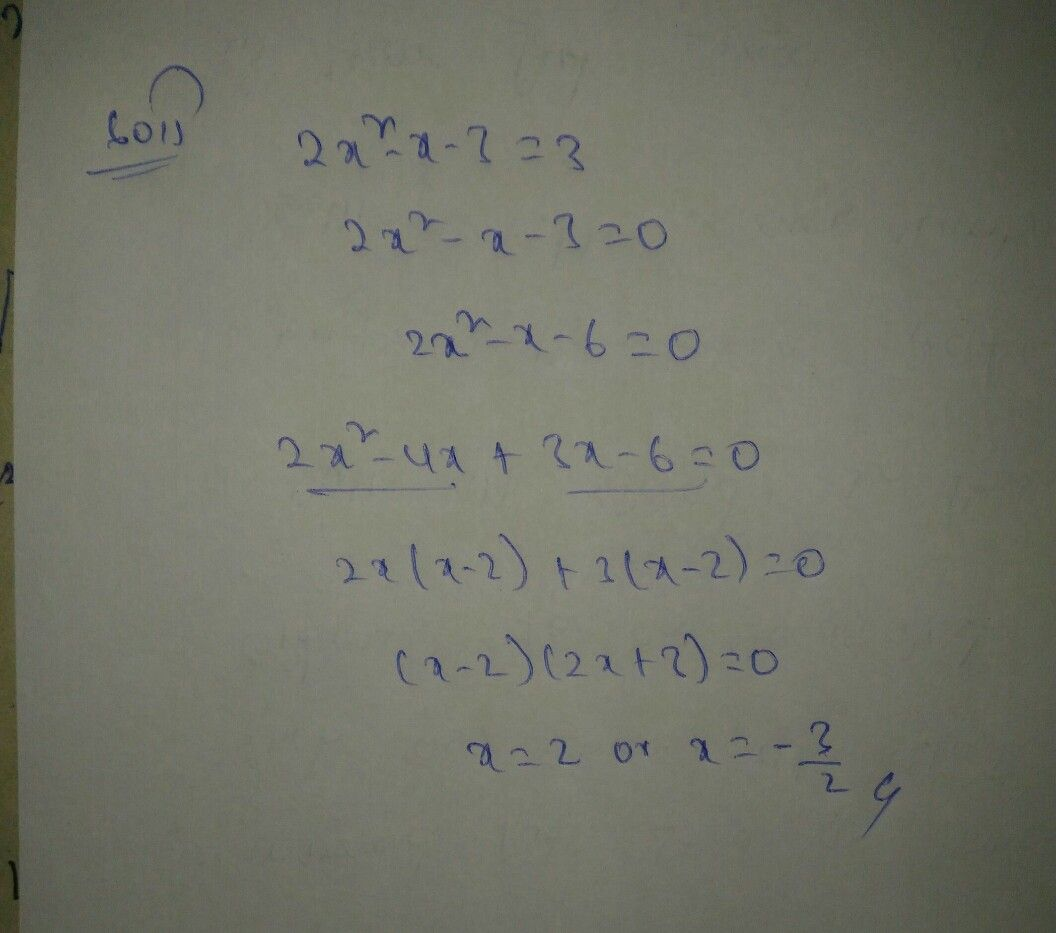Symbol
ProblemSolve for x: $2x^{2}-x-3=3$ $2x^{2}-x-3-3=0$
Trigonometry
SolutionQanda teacher - chandu